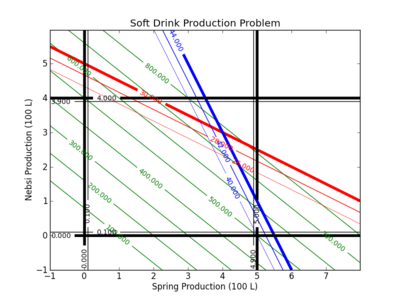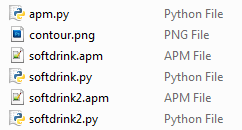### Linear Programming Example

A refinery must produce 100 gallons of gasoline and 160 gallons of diesel to meet customer demands. The refinery would like to minimize the cost of crude and two crude options exist. The less expensive crude costs $80 USD per barrel while a more expensive crude costs$95 USD per barrel. Each barrel of the less expensive crude produces 10 gallons of gasoline and 20 gallons of diesel. Each barrel of the more expensive crude produces 15 gallons of both gasoline and diesel. Find the number of barrels of each crude that will minimize the refinery cost while satisfying the customer demands.

#### Soft Drink Production Problem (Example 2)

A simple production planning problem is given by the use of two ingredients A and B that produce products 1 and 2. The available supply of A is 30 units and B is 44 units. For production it requires:

• 3 units of A and 8 units of B to produce Product 1
• 6 units of A and 4 units of B to produce Product 2

There are at most 5 units of Product 1 and 4 units of Product 2. Product 1 can be sold for 100 and Product 2 can be sold for 125. The objective is to maximize the profit for this production problem.

#### Python Solution

from gekko import GEKKO

m = GEKKO()
x1 = m.Var(lb=0,ub=5)
x2 = m.Var(lb=0,ub=4)
profit = m.Var()

m.Maximize(profit) # maximize
m.Equation(profit==100*x1 + 125*x2)
m.Equation(3*x1+6*x2<=30)
m.Equation(8*x1+4*x2<=44)

m.solve()

print ('')
print ('--- Results of the Optimization Problem ---')
print ('Product 1 (x1): ' + str(x1.value))
print ('Product 2 (x2): ' + str(x2.value))
print ('Profit: ' + str(profit.value))

#### Contour Plot

A contour plot can be used to explore the optimal solution. In this case, the black lines indicate the upper and lower bounds on the production of 1 and 2. In this case, the production of 1 must be greater than 0 but less than 5. The production of 2 must be greater than 0 but less than 4.#### Solution and Contour Plots with Python

Below are the source files for generating the contour plots in GEKKO Python and APM Python.

from gekko import GEKKO

m = GEKKO()
x1 = m.Var(lb=0,ub=5)
x2 = m.Var(lb=0,ub=4)
profit = m.Var()

m.Maximize(profit)
m.Equation(profit==100*x1 + 125*x2)
m.Equation(3*x1+6*x2<=30)
m.Equation(8*x1+4*x2<=44)

m.solve()

print ('')
print ('--- Results of the Optimization Problem ---')
print ('Product 1 (x1): ' + str(x1.value))
print ('Product 2 (x2): ' + str(x2.value))
print ('Profit: ' + str(profit.value))

## Generate a contour plot
# Import some other libraries that we'll need
# matplotlib and numpy packages must also be installed
import matplotlib
import numpy as np
import matplotlib.pyplot as plt

# Design variables at mesh points
x = np.arange(-1.0, 8.0, 0.02)
y = np.arange(-1.0, 6.0, 0.02)
x1, x2 = np.meshgrid(x,y)

# Equations and Constraints
profit = 100.0 * x1 + 125.0 * x2
A_usage = 3.0 * x1 + 6.0 * x2
B_usage = 8.0 * x1 + 4.0 * x2

# Create a contour plot
plt.figure()
# Weight contours
lines = np.linspace(100.0,800.0,8)
CS = plt.contour(x1,x2,profit,lines,colors='g')
plt.clabel(CS, inline=1, fontsize=10)
# A usage < 30
CS = plt.contour(x1,x2,A_usage,[26.0, 28.0, 30.0],colors='r',linewidths=[0.5,1.0,4.0])
plt.clabel(CS, inline=1, fontsize=10)
# B usage < 44
CS = plt.contour(x1, x2,B_usage,[40.0,42.0,44.0],colors='b',linewidths=[0.5,1.0,4.0])
plt.clabel(CS, inline=1, fontsize=10)
# Container for 0 <= Product 1 <= 500 L
CS = plt.contour(x1, x2,x1 ,[0.0, 0.1, 4.9, 5.0],colors='k',linewidths=[4.0,1.0,1.0,4.0])
plt.clabel(CS, inline=1, fontsize=10)
# Container for 0 <= Product 2 <= 400 L
CS = plt.contour(x1, x2,x2 ,[0.0, 0.1, 3.9, 4.0],colors='k',linewidths=[4.0,1.0,1.0,4.0])
plt.clabel(CS, inline=1, fontsize=10)

# Add some labels
plt.title('Soft Drink Production Problem')
plt.xlabel('Product 1 (100 L)')
plt.ylabel('Product 2 (100 L)')
# Save the figure as a PNG
plt.savefig('contour.png')

# Show the plots
plt.show()

The linear program is solved with the APM model through a web-service while the contour plot is generated with the Python package Matplotlib.# Import APM Python library
try:
from APMonitor import *
except:
# Automatically install APMonitor
import pip
pip.main(['install','APMonitor'])
from APMonitor import *

# Select the server
server = 'https://byu.apmonitor.com'

# Give the application a name
app = 'production'

# Clear any previous applications by that name
apm(server,app,'clear all')

# Write the model file
fid = open('softdrink.apm','w')
fid.write('Variables \n')
fid.write('  x1 > 0 , < 5  ! Product 1 \n')
fid.write('  x2 > 0 , < 4  ! Product 2 \n')
fid.write('  profit \n')
fid.write(' \n')
fid.write('Equations \n')
fid.write('  ! profit function \n')
fid.write('  maximize profit   \n')
fid.write('  profit = 100 * x1 + 125 * x2 \n')
fid.write('  3 * x1 + 6 * x2 <= 30 \n')
fid.write('  8 * x1 + 4 * x2 <= 44         \n')
fid.close()

# Solve on APM server
solver_output = apm(server,app,'solve')

# Display solver output
print(solver_output)

# Retrieve results
sol = apm_sol(server,app)

print ('')
print ('--- Results of the Optimization Problem ---')
print ('Product 1 (x1): ' + str(sol['x1']))
print ('Product 2 (x2): ' + str(sol['x2']))
print ('Profit: ' + str(sol['profit']))

# Display Results in Web Viewer
url = apm_web_var(server,app)

## Generate a contour plot
# Import some other libraries that we'll need
# matplotlib and numpy packages must also be installed
import matplotlib
import numpy as np
import matplotlib.pyplot as plt

# Design variables at mesh points
x = np.arange(-1.0, 8.0, 0.02)
y = np.arange(-1.0, 6.0, 0.02)
x1, x2 = np.meshgrid(x,y)

# Equations and Constraints
profit = 100.0 * x1 + 125.0 * x2
A_usage = 3.0 * x1 + 6.0 * x2
B_usage = 8.0 * x1 + 4.0 * x2

# Create a contour plot
plt.figure()
# Weight contours
lines = np.linspace(100.0,800.0,8)
CS = plt.contour(x1,x2,profit,lines,colors='g')
plt.clabel(CS, inline=1, fontsize=10)
# A usage < 30
CS = plt.contour(x1,x2,A_usage,[26.0, 28.0, 30.0],colors='r',linewidths=[0.5,1.0,4.0])
plt.clabel(CS, inline=1, fontsize=10)
# B usage < 44
CS = plt.contour(x1, x2,B_usage,[40.0,42.0,44.0],colors='b',linewidths=[0.5,1.0,4.0])
plt.clabel(CS, inline=1, fontsize=10)
# Container for 0 <= Product 1 <= 500 L
CS = plt.contour(x1, x2,x1 ,[0.0, 0.1, 4.9, 5.0],colors='k',linewidths=[4.0,1.0,1.0,4.0])
plt.clabel(CS, inline=1, fontsize=10)
# Container for 0 <= Product 2 <= 400 L
CS = plt.contour(x1, x2,x2 ,[0.0, 0.1, 3.9, 4.0],colors='k',linewidths=[4.0,1.0,1.0,4.0])
plt.clabel(CS, inline=1, fontsize=10)

# Add some labels
plt.title('Soft Drink Production Problem')
plt.xlabel('Product 1 (100 L)')
plt.ylabel('Product 2 (100 L)')
# Save the figure as a PNG
plt.savefig('contour.png')

# Show the plots
plt.show()